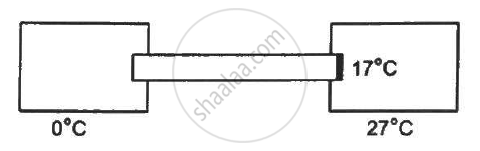Share

# A Cylindrical Rod of Length 50 Cm and Cross Sectional Area 1 Cm2 is Fitted Between a Large Ice Chamber at 0°C and an Evacuated Chamber Maintained at 27°C as - Physics

#### Question

A cylindrical rod of length 50 cm and cross sectional area 1 cm2 is fitted between a large ice chamber at 0°C and an evacuated chamber maintained at 27°C as shown in the figure. Only small portions of the rod are inside the chamber and the rest is thermally insulated from the surrounding. The cross section going into the evacuated chamber is blackened so that it completely absorbs any radiation falling on it. The temperature of the blackened end is 17°C when steady state is reached. Stefan constant σ = 6 × 10−8 W m−2 K−4. Find the thermal conductivity of the material of the rod.#### Solution

Length, l = 50 cm

Cross sectional area, A =1 cm2

Stefan's constant, σ = 6 × 10−8 W m−2 K−4

Temperature of the blackened end = 17°C

Temperature of the chamber =  27°C

Temperature of one end of the rod = 0°C

According to Stefan's law,

(DeltaQ)/(Deltat) = ε. A σ (T_2^4 - T_1^4)

(DeltaQ)/(Deltat.A) =ε σ (T_2^4 - T_1^4)

(DeltaQ)/(Deltat.A) = 1xx6xx10^-s ((300)^4 - (290)^4)

= 6 × 10.3                  .... (1)

Also , (Delta Q)/(Delta t) = (KA(T_1 - T_2))/l   ........(2)

From (1) and (2),
6 × 10.3 = (K × 17)/0.5`
k = 1.8 w/ °C

Is there an error in this question or solution?

#### APPEARS IN

Solution A Cylindrical Rod of Length 50 Cm and Cross Sectional Area 1 Cm2 is Fitted Between a Large Ice Chamber at 0°C and an Evacuated Chamber Maintained at 27°C as Concept: Heat Transfer - Radiation.
S